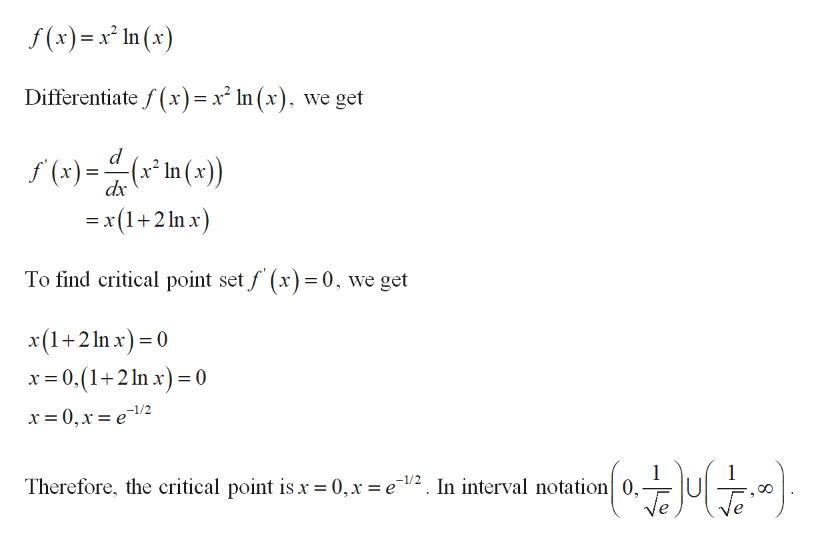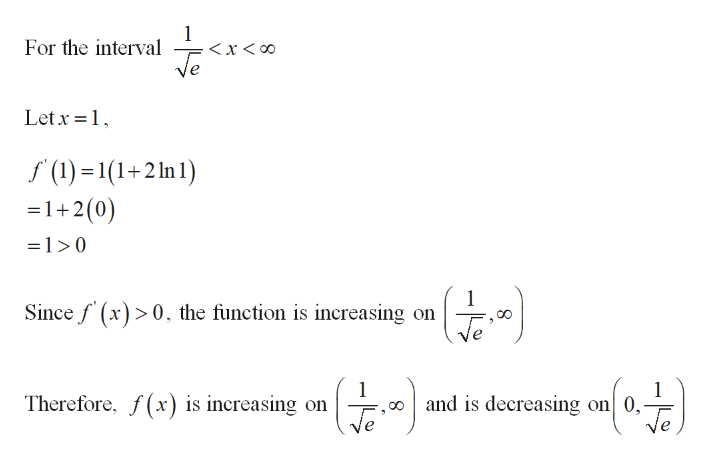# Consider the following function:f(x)=x^2ln(x)a.) find the intervals on which f is increasing or decreasingb.) find local maximum and minimum valuesc.) find intervals of concavity and inflection points

Question
33 views

Consider the following function:

f(x)=x^2ln(x)

a.) find the intervals on which f is increasing or decreasing

b.) find local maximum and minimum values

c.) find intervals of concavity and inflection points

check_circle

Step 1

a)

Consider the function,help_outlineImage Transcriptionclosef (x) x In (x) Differentiate f (x)= x2 In (x), we get d (x2 In (x)) x(1+2 In x) To find critical point set f (x) = 0, we get x(1+2 n x)( x0,(12 n x 0 x = 0,x =e1/2 Therefore, the critical point is x = 0,x = e12. In interval notation 0, ((F»} fullscreen
Step 2
Step 3help_outlineImage Transcriptionclose1 x oo For the interval Let x 1 (1)1(1+2 In 1) =1+2(0) =1>0 1 Sincef (x)0 the function is increasing on («4) ,aoand is decreasing on 0, Therefore, f(x is increasing on fullscreen

### Want to see the full answer?

See Solution

#### Want to see this answer and more?

Solutions are written by subject experts who are available 24/7. Questions are typically answered within 1 hour.*

See Solution
*Response times may vary by subject and question.
Tagged in

### Calculus